Computer Science TopicsConditional ProbabilityMachine Learning

# Derivative of the Sigmoid functionSigmoid and Dino

In this article, we will see the complete derivation of the Sigmoid function as used in Artificial Intelligence Applications.

To start with, let’s take a look at the sigmoid functionSigmoid function

Okay, looks sweet!
We read it as, the sigmoid of x is 1 over 1 plus the exponential of negative x.
And this is the equation (1).

Let’s take a look at the graph of the sigmoid function,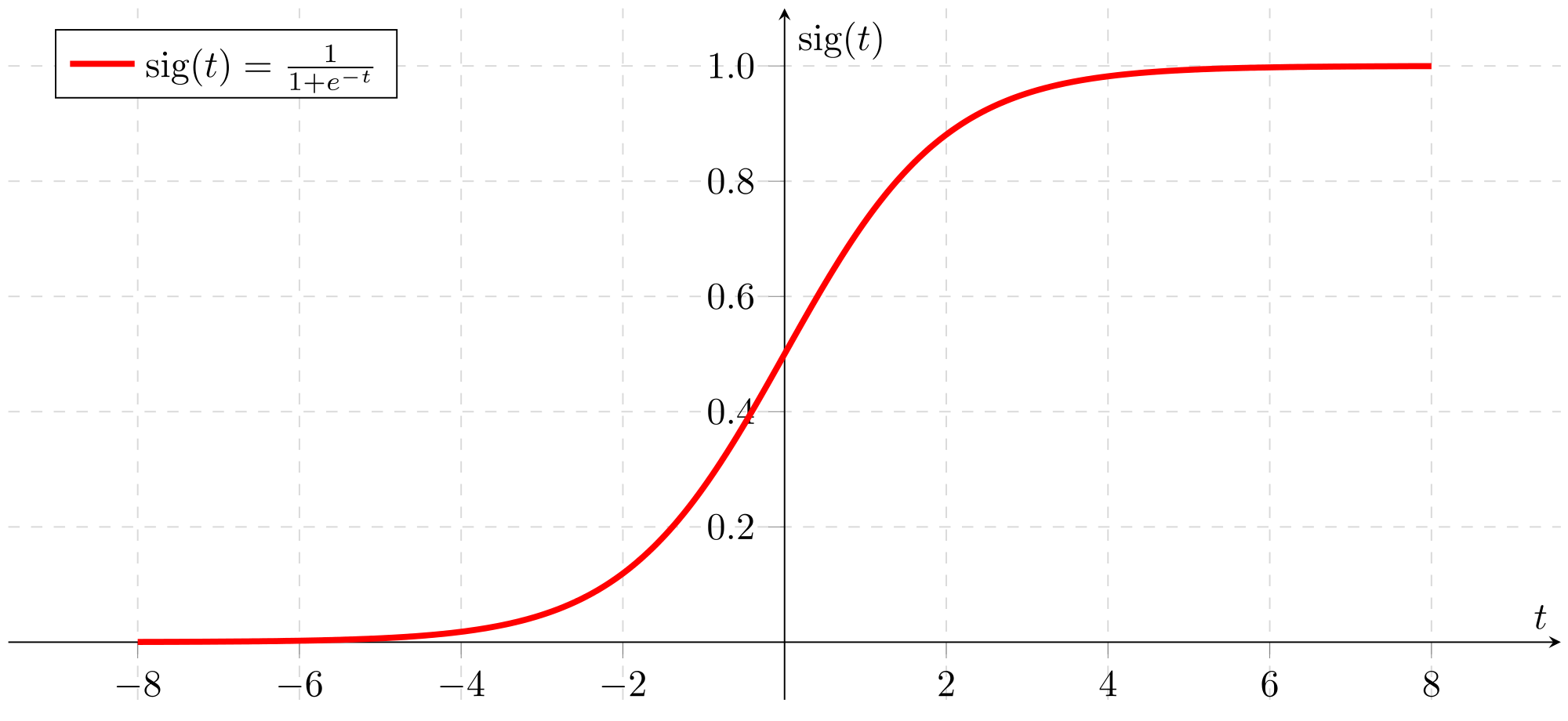Graph of the Sigmoid Function

Looking at the graph, we can see that the given a number `n`, the sigmoid function would map that number between 0 and 1.
As the value of `n` gets larger, the value of the sigmoid function gets closer and closer to and as `n` gets smaller, the value of the sigmoid function is get closer and closer to 0.

Okay, so let’s start deriving the sigmoid function!
So, we want the value of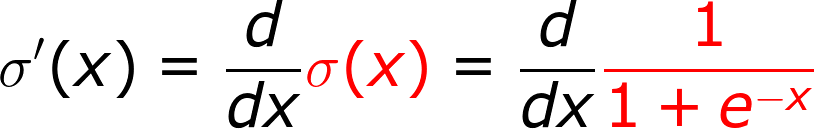Step 1

In the above step, I just expanded the value formula of the sigmoid function from (1)

Next, let’s simply express the above equation with negative exponents,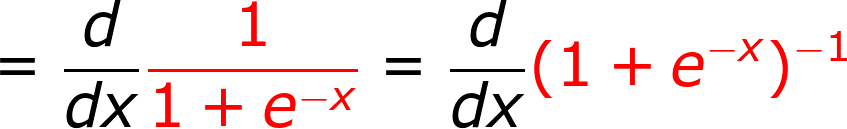Step 2

Next, we will apply the reciprocal rule, which simply saysReciprocal Rule

Applying the reciprocal rule, takes us to the next step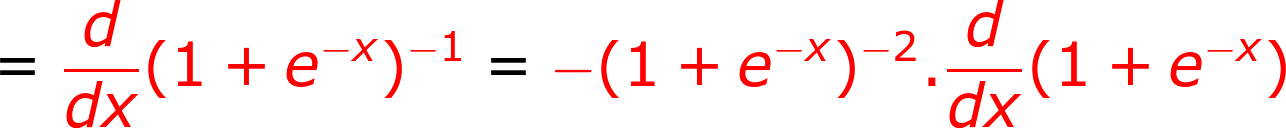Step 3

To clearly see what happened in the above step, replace `u(x)` in the reciprocal rule with `(1 + e^(-x))` .

Next, we need to apply the rule of linearity, which simply says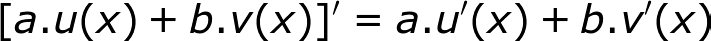Rule of Linearity

Applying the rule of linearity, we get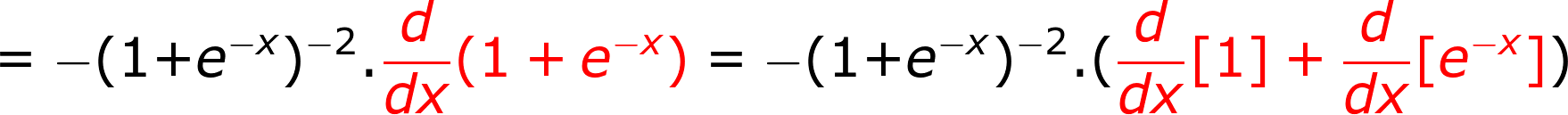Step 4

Okay, that was simple, now let’s derive each of them one by one.
Now, derivative of a constant is 0, so we can write the next step asStep 5

And adding 0 to something doesn’t effects so we will be removing the 0 in the next step and moving with the next derivation for which we will require the exponential rule, which simply saysExponential Rule

Applying the exponential rule we get,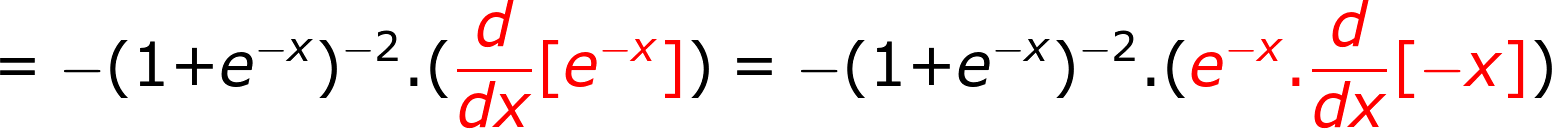Step 6

Again, to better understand you can simply replace `e^u(x)` in the exponential rule with `e^(-x)`

Next, by the rule of linearity we can write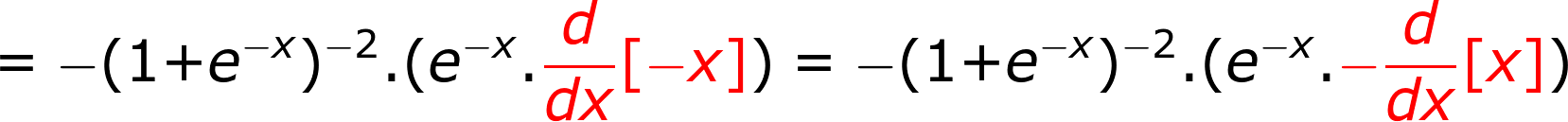Step 7

Derivative of the differentiation variable is 1, applying which we getStep 8

Now, we can simply open the second pair of parenthesis and applying the basic rule `-1 * -1 = +1` we getStep 9

which can be written as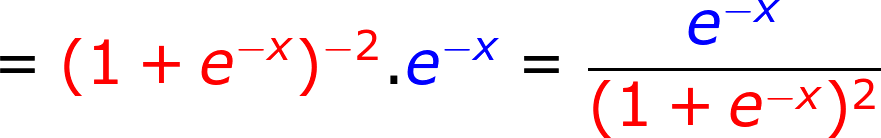Step 10

Okay, we are complete with the derivative!!

But but but, we still need to simplify it a bit to get to the form used in Machine Learning. Okay, let’s go!

First, let’s rewrite it as follows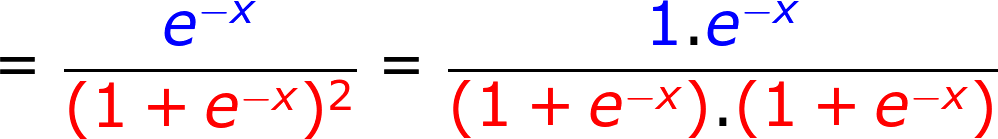Step 11

And then rewrite it as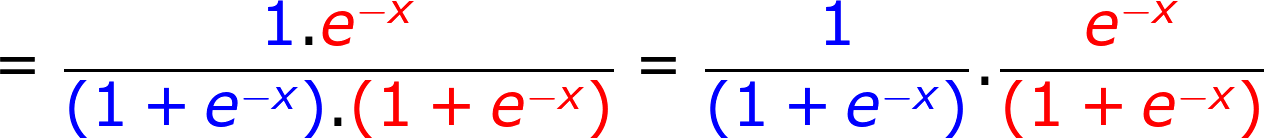Step 12

And since `+1 — 1 = 0` we can do this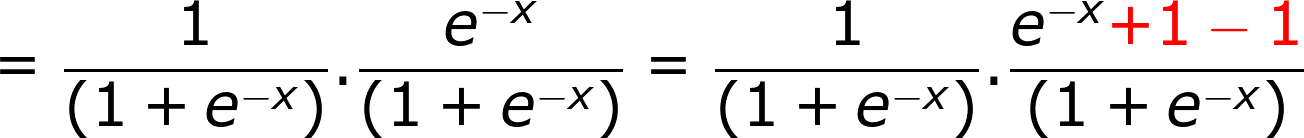Step 13

And now let’s break the fraction and rewrite it as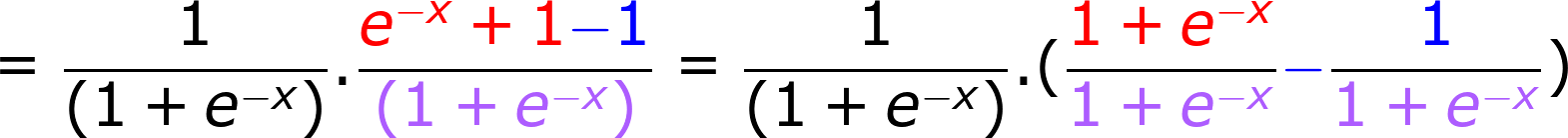Step 14

Let’s cancel out the numerator and denominatorStep 15

Now, if we take a look at the first equation of this article (1), then we can rewrite as follows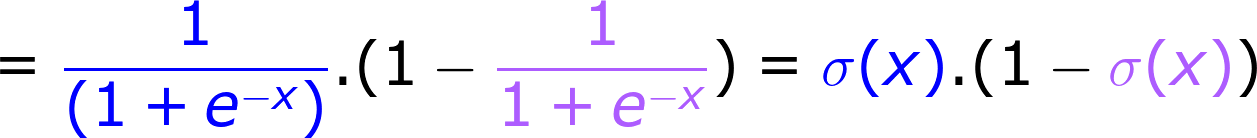Step 16

And with that the simplification is complete!

So, the derivative of the sigmoid function isDerivative of the Sigmoid Function

And the graph of the derivative of the sigmoid function looks like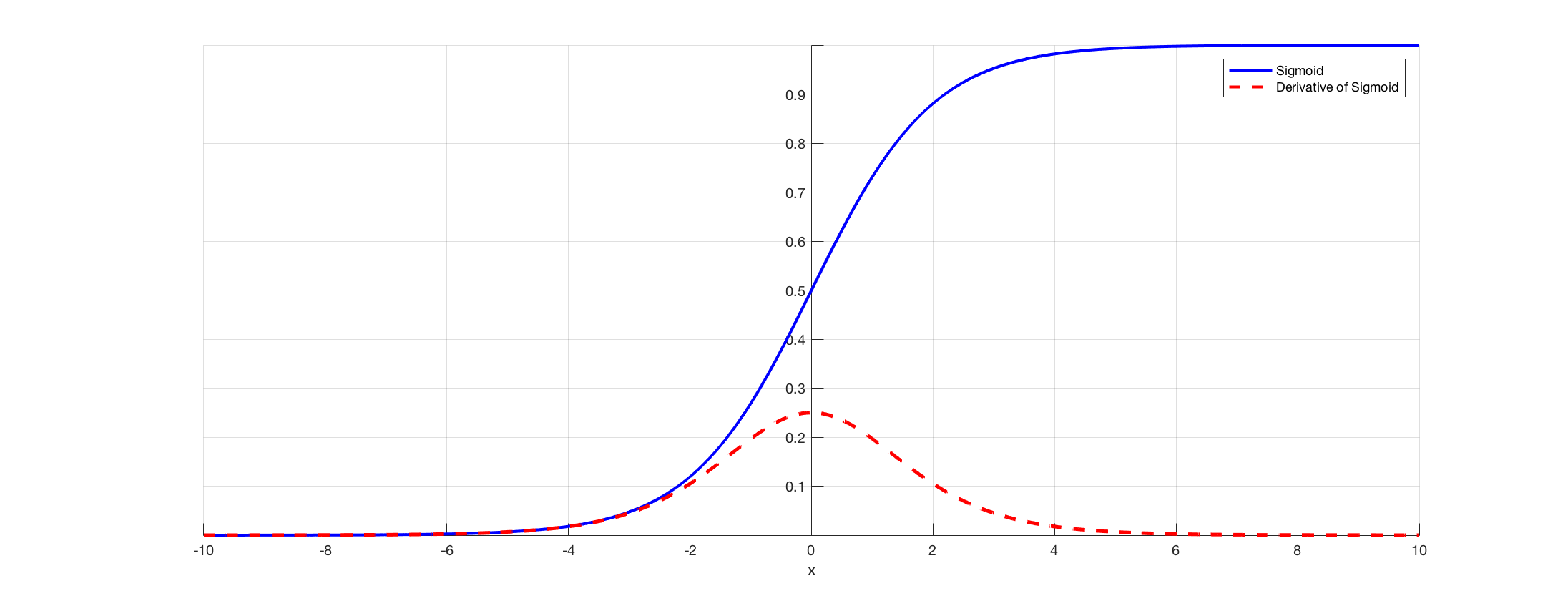Graph of Sigmoid and the derivative of the Sigmoid function
Previous Post

Next article